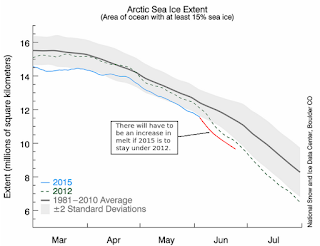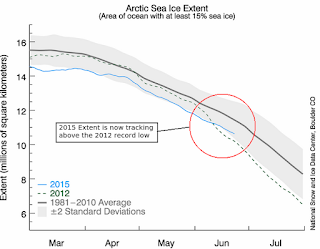## Thursday, June 11, 2015

### The Question Is: How Much Acceleration Is Involved In SLR - 7?Fig. 1 2015 Lowest Year Early-On
This year has seen early low Arctic Sea Ice levels (Fig. 1).

It is at a point where the trend line is showing that, without an acceleration in the 2015 current melt rate, the 2012 level will (at least temporarily) take first place again.

And it looks like that could happen in a few days.

That is, if 2015 stays on its current trajectory, this year's melt rate will result in a drop below the 2012 rate, which is the current historical record low year for Arctic Sea Ice cover.

In Fig. 2 the current trend is shown by the light blue line, then is projected out (red line) to where it is going without any acceleration.Fig. 2 Trend line projection

In Fig. 3 the required acceleration is shown as a red line curving below the 2012 melt rate at this time of year back then.

So, it remains to be seen whether the 2015 melt rate will increase to stay in the lead.

In this series I have tried to make the point that the rate of acceleration varies all the time, like traffic at an intersection with congestion.

Some times the traffic moves slower, sometimes it accelerates, but the unknown is when each will happen.Fig. 3 Acceleration Needed to beat 2012
Once it happens it is easier to calculate how long it will take to finally get through the jam.

In the present scenario, the 2015 melt will have to imitate the 2012 pattern.

That would require an acceleration at this time, as the 2012 season did at this time (Fig. 3).

All that can be said is that it is not clear which way things will go in June because there are some cooler trends and some warmer trends, each of which could play the deciding role in causing acceleration or causing a slow down in the melt.

Let's review the impact of acceleration in terms of the Hansen 5, 7, and 10 year  doubling (acceleration), and the Dredd Blog sea level rise (SLR) 3 yr. doubling model (The Evolution of Models - 10).

And for grins, the 2 yr. doubling (The Question Is: How Much Acceleration Is Involved In SLR - 5?).Acceleration per NOAA

The effect that 2, 3, 5, 7, and 10 year doubling has is that it determines when SLR reaches the 1 m / 3 ft. level:
10 yr = 2067
7 yr = 2055
5 yr = 2045
3 yr = 2035
2 yr = 2031
(The Question Is: How Much Acceleration Is Involved In SLR - 5?).  We focus most on 1 m / 3ft. because it is a point where many locations that are tidal will experience serious problems, especially with coastal infrastructure and ports (The 1% May Face The Wrath of Sea Level Rise First).

So, what does sea ice have to do with it?

The Arctic will warm faster (acceleration) when the ice cover melts and the darker ocean begins to absorb sunlight in contrast to the ice reflecting sunlight.

And that is why we watch the sea ice cover melt rate to see if 2015 will take the lead from 2012 as the year closest to the year when there is no ice cover on the Arctic Ocean.Fig. 4 2015 compared to 2012
UPDATES: On about June 13, the 2015 extent line increased above the 2012 extent line as projected (see Fig. 2).

This year's tracking of the Arctic Ice Extent is here.

As of about June 22, 2015, the extent continued as projected in Fig. 2 (compare Fig. 4).

Basically this year is oscillating back and forth between being the lowest ice extent on record, to then falling back behind 2012 (which has been the record low extent year so far).

Notice that the heading of the NSIDC graph is entitled "Arctic Sea Ice Extent (Area of ocean with at least 15% sea ice)" because "extent" and "area" mean the same thing mathematically (a = l * w or  e = l * w).

Extent and area do not indicate how much ice there is, only how much ocean is covered, so they are expressed in km2 or mi2, while the quantity of ice is called "volume" (v = l * w * h) and is expressed as km3 or mi3 (How Fifth Graders Calculate Ice Volume, 2, 3, 4).

Whether 2015 or 2012 will be the record low extent looks like a toss-up at this point.

The next post in this series is here, the previous post in this series is here.

#### 1 comment:

1.2031 to 2067 is a 36 year difference.

I'd say rate of acceleration is a big deal.